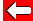# Adding Fractions

Now you can find the common denominator of fractions, we can do sums with them! Let's try 1/2 + 1/4.

First get them common denominators: 2/4 + 1/4

Then add their numerators: 2 + 1 = 3.

Full calculation: 1/2 + 1/4 = 2/4 + 1/4 = 3/4

Subtracting fractions can be done in the same way.Go back a pageMaths MenuGo to next page